Next: Neutron drip line Up: Examples Previous: Self-consistent calculation for Sn

## Regularization of the pairing field

In this section, we present results obtained by using the regularization method proposed by A. Bulgac and Y. Yu . Calculations have been made with the same box size and integration step as in the previous section, and the partial waves have been included up to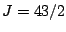. The effective force used is SLy4, see table 1, combined with the mixed pairing force with parameters given in table 2. When evaluating the densities, contributions of quasiparticle states are included up to the maximum equivalent energy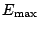, see Eq. (47), but once this maximum energy is high enough the global properties of the nucleus do not depend on it.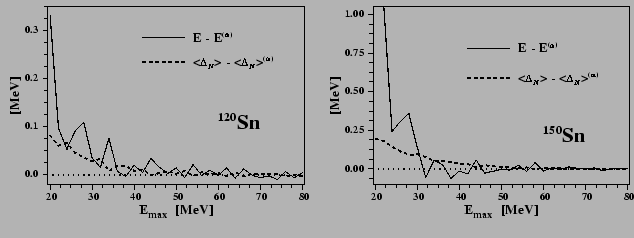It appears that the stability of results is very satisfying, even for a very exotic nucleus. This is shown in Figure 6, where the total energy and mean neutron gap are displayed as functions offor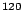Sn and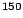Sn. Forgreater than 60 MeV, where the total energy does not show any significant evolution, we have evaluated its asymptotic limit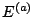, and the analogous limit of the neutron mean pairing gap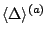, by averaging their respective values over the interval ranging from 60 to 80 MeV. The results are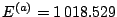MeV and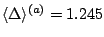MeV forSn, and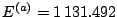MeV and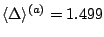MeV forSn. In this interval, the energies are scattered within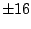keV and the gaps within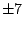keV. Since the increase offrom 60 MeV to 80 MeV does not change the results in a significant way, the choice of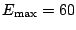MeV has been made for the rest of this study. In principle, this value should be readjusted in other mass region or when using a different effective force.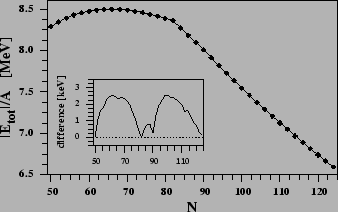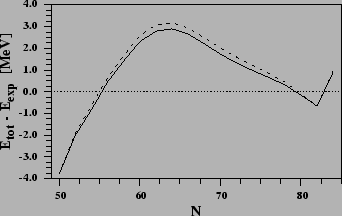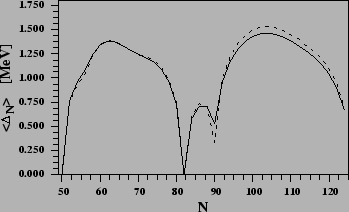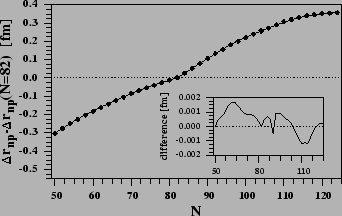Within the cut-off prescription and regularization scheme we have calculated the series of even-even tin isotopes by using the SLy4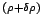force. The left part of Fig. 7 displays the binding energies per particle and the right part the deviation between the calculated binding energies and the experimental ones . One can see that both methods give very similar results. The neutron mean gap are plotted on the left part of Fig. 8. In the two sets of calculation, the strengths of the pairing force have been adjusted in order to give the same gap inSn. Again, we do not observe here any significant change when using or not the regularization scheme, although the gap is slightly reduced in heavy tin isotopes. Finally, the right part of Fig. 8 compares the differences between the neutron and proton rms radii plotted with respect to that in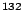Sn; once again the two methods give extremely similar results.Next: Neutron drip line Up: Examples Previous: Self-consistent calculation for Sn
Jacek Dobaczewski 2005-01-23